Functions and Algebra: Sketch and interpret functions and graphs

# Unit 5: Trigonometric functions

Dylan Busa### Unit outcomes

By the end of this unit you will be able to:

• Identify the following characteristics of trigonometric functions:
• periodicity
• amplitude.
• Sketch the graph $\scriptsize y=a\sin x+q$, $\scriptsize y=a\cos x+q$ and $\scriptsize y=a\tan x+q$.
• Identify the asymptotes of $\scriptsize y=a\tan x+q$.
• Investigate and generalise the impact of $\scriptsize a$ and $\scriptsize q$ on $\scriptsize y=asinx+q$, $\scriptsize y=a\cos x+q$ and $\scriptsize y=a\tan x+q$.

## What you should know

Before you start this unit, make sure you can

## Introduction

All modern music is produced with the aid of computers at some stage in the recording and mastering process. Computers are used to manipulate, transform and blend the sounds made by instruments of various kinds. These computer programmes use trigonometric functions to help them model and change sounds.

When sound travels through the air, the energy of the sound creates pockets of air molecules at slightly different pressures (see Figure 1). In some of these areas the air is slightly compressed and at a higher pressure than normal. In others, it is decompressed and at a lower pressure.

These sound waves can be thought of in the same way as waves travelling across a pond, where the areas of compression correspond to the crests or peaks and the areas of decompression correspond to the troughs or valleys (see Figure 2).

These waves can be modelled mathematically using a trigonometric function called sine. If we can model the wave using a function, it means we can manipulate it in all sorts of ways. Computers, being particularly good at maths, can make very complicated changes to very complex waves quickly and easily.

This is just one example of where trigonometric functions are used in real life. Another example is an engineer or architect calculating forces that are at an angle that will be experienced by physical structures like buildings or bridges.

In this unit, we will explore the trigonometric functions called sine ($\scriptsize \sin$), cosine ($\scriptsize \cos$) and tangent ($\scriptsize \tan$). All three functions are based on how the ratios of the length of sides in a right-angled triangle change as the angle inside the triangle changes. Figure 3 shows a summary.

• $\scriptsize \sin \theta$ is the ratio of the length of the side opposite the angle $\scriptsize \theta$ and the hypotenuse (the side opposite the right angle). Another way of saying this is that it is the value of the y-coordinate, of the point made by the radius that is $\scriptsize r$ units long and that is $\scriptsize {{\theta }^{{}^\circ }}$ away from the positive x-axis, divided by $\scriptsize r$.
• $\scriptsize \cos \theta$ is the ratio of the length of the side adjacent (next to) the angle $\scriptsize \theta$ and the hypotenuse. Another way of saying this is that it is the value of the x-coordinate, of the point made by the radius that is $\scriptsize r$ units long and that is $\scriptsize {{\theta }^{{}^\circ }}$ away from the positive x-axis, divided by $\scriptsize r$.
•  $\scriptsize \tan \theta$ is the ratio of the length of the side opposite the angle $\scriptsize \theta$ and the length of the side adjacent to the angle. Another way of saying this is that it is the value of the y-coordinate, of the point made by the radius that is $\scriptsize r$ units long and that is $\scriptsize {{\theta }^{{}^\circ }}$ away from the positive x-axis, divided by the x-coordinate of this point.

### Note

If you need more help understanding these three basic trigonometric ratios, watch the introductory video called “Basic trigonometry” or complete Subject outcome 3.6, Unit 1: Trigonometric ratios before continuing with this unit.

Basic trigonometry (Duration: 9.16)# The sine and cosine functions

In this unit, we will deal with the sine and cosine functions together because they are very similar. As we will see, the cosine function is really just the sine function shifted horizontally by $\scriptsize {{90}^{{}^\circ }}$.### Activity 5.1: Investigate the sine and cosine functions

Time required: 60 minutes

What you need:

• a pen or pencil
• a calculator
• blank paper or a notebook
• a ruler
• a protractor
• an internet connection (highly recommended)

What to do:

Part A

1. On a piece of paper, draw a large Cartesian plane. Each axis should be at least $\scriptsize 15\ \text{cm}$ long.
2. Now put your protractor on the origin and measure an angle of $\scriptsize {{30}^{{}^\circ }}$ from the positive x-axis and mark this point. Draw a line $\scriptsize 10\ \text{cm}$ long from the origin going through the point you marked off. We will call this line the radius.
3. Draw a perpendicular line from the end of the radius to the x-axis to create a right-angled triangle with $\scriptsize {{30}^{{}^\circ }}$ at the origin.
4. Create a table like this on another piece of paper.
 $\scriptsize \theta$ $\scriptsize {{30}^{{}^\circ }}$ $\scriptsize {{45}^{{}^\circ }}$ $\scriptsize {{60}^{{}^\circ }}$ $\scriptsize {{90}^{{}^\circ }}$ $\scriptsize {{120}^{{}^\circ }}$ $\scriptsize {{135}^{{}^\circ }}$ $\scriptsize {{150}^{{}^\circ }}$ $\scriptsize {{180}^{{}^\circ }}$ $\scriptsize {{210}^{{}^\circ }}$ $\scriptsize {{240}^{{}^\circ }}$ $\scriptsize {{270}^{{}^\circ }}$ $\scriptsize {{300}^{{}^\circ }}$ $\scriptsize {{330}^{{}^\circ }}$ $\scriptsize {{360}^{{}^\circ }}$ $\scriptsize y$ $\scriptsize \displaystyle \frac{y}{r}$
5. Measure the length of the side opposite the $\scriptsize {{30}^{{}^\circ }}$ angle. This gives you the y-coordinate of the point made by the end of the radius. Write this in the table under the $\scriptsize {{30}^{{}^\circ }}$.
6. Now measure an angle of $\scriptsize {{45}^{{}^\circ }}$ from the positive x-axis, draw another radius $\scriptsize 10\ \text{cm}$ long, drop a perpendicular line, measure the length of this perpendicular line to determine the y-coordinate of the point at the end of the radius and fill it into your table.
7. Complete the rest of the table in the same way. Just remember that when the y-coordinate at the end of your radius is below the x-axis, you need to show that this is a negative value in your table.
8. Once the second row of your table is complete, calculate the values in the third row. Remember that in every case $\scriptsize r=10$.
9. Now draw a Cartesian plane like the one in Figure 4 and plot the points from your table on it.
10. Finally, join these points with as smooth a ‘wavy’ curve as you can.

You have just drawn the sine function $\scriptsize f(\theta )=\sin \theta$ for $\scriptsize {{0}^{{}^\circ }}\le \theta \le {{360}^{{}^\circ }}$. You plotted the value of the ratio $\scriptsize \displaystyle \frac{y}{r}$ as $\scriptsize \theta$ increased from $\scriptsize {{0}^{{}^\circ }}$ to $\scriptsize {{360}^{{}^\circ }}$.

11. Now answer these questions about the graph you have just drawn.
1. What is the maximum value the function reaches? What is the minimum value?
2. What are the turning points of the graph?
3. What do you think happens to the function if we continue past $\scriptsize {{360}^{{}^\circ }}$ or to the left of $\scriptsize {{0}^{{}^\circ }}$?
4. What is the domain and range of the function $\scriptsize f(\theta )=\sin \theta$?

Part B

1. Make a copy of this table on a piece of paper
 $\scriptsize \theta$ $\scriptsize {{30}^{{}^\circ }}$ $\scriptsize {{45}^{{}^\circ }}$ $\scriptsize {{60}^{{}^\circ }}$ $\scriptsize {{90}^{{}^\circ }}$ $\scriptsize {{120}^{{}^\circ }}$ $\scriptsize {{135}^{{}^\circ }}$ $\scriptsize {{150}^{{}^\circ }}$ $\scriptsize {{180}^{{}^\circ }}$ $\scriptsize {{210}^{{}^\circ }}$ $\scriptsize {{240}^{{}^\circ }}$ $\scriptsize {{270}^{{}^\circ }}$ $\scriptsize {{300}^{{}^\circ }}$ $\scriptsize {{330}^{{}^\circ }}$ $\scriptsize {{360}^{{}^\circ }}$ $\scriptsize x$ $\scriptsize \displaystyle \frac{x}{r}$
2. Go back to your first Cartesian plane and now measure the length of the line adjacent to the angle $\scriptsize \theta$ in each case. Remember, the adjacent line is the line next to the angle that is not the radius. Fill these values into the second row of your table.
3. Calculate the values in the third row of the table.
4. Now draw a Cartesian plane like the one in Figure 4 and plot the points from your table on it.
5. Finally, join these points with as smooth a ‘wavy’ curve as you can. You have just drawn the cosine function $\scriptsize f(\theta )=\cos \theta$ for $\scriptsize {{0}^{{}^\circ }}\le \theta \le {{360}^{{}^\circ }}$. You plotted the value of the ratio $\scriptsize \displaystyle \frac{x}{r}$ as $\scriptsize \theta$ increased from $\scriptsize {{0}^{{}^\circ }}$ to $\scriptsize {{360}^{{}^\circ }}$.
6. Now answer these question about the graph you have just drawn.
1. What is the maximum value the function reaches? What is the minimum value?
2. What are the turning points of the graph?
3. What do you think happens to the function if we continue past $\scriptsize {{360}^{{}^\circ }}$ or to the left of $\scriptsize {{0}^{{}^\circ }}$?
4. What is the domain and range of the function $\scriptsize f(\theta )=\cos \theta$?

Part C

1. When you have an internet connection, visit the sine function interactive simulation.Here you will see a unit circle similar to the one you created in Part A and a slider with which you can change the value of the angle $\scriptsize \theta$. Drag the slider and notice how the function value of the graph of $\scriptsize f(\theta )=\sin \theta$ always tracks the length of the side opposite the angle.
2. Now visit the cosine function interactive simulation.Here you will find a unit circle similar to the one you used in Part B and a slider with which you can change the value of the angle $\scriptsize \theta$. Drag the slider and notice how the function value of the graph of $\scriptsize f(\theta )=\cos \theta$ always tracks the length of the side adjacent to the angle.

What did you find?

Part A

Figure 5 shows what the various triangles you drew should look like. The figure indicates the lengths of the sides opposite the angle as well as the x- and y-coordinates of the points formed by the radius and the angle. We call a circle like this a unit circle. In our case, $\scriptsize 10\ \text{cm = 1 unit}$.

Table 1 shows what your completed table should look like.

 $\scriptsize \theta$ $\scriptsize {{30}^{{}^\circ }}$ $\scriptsize {{45}^{{}^\circ }}$ $\scriptsize {{60}^{{}^\circ }}$ $\scriptsize {{90}^{{}^\circ }}$ $\scriptsize {{120}^{{}^\circ }}$ $\scriptsize {{135}^{{}^\circ }}$ $\scriptsize {{150}^{{}^\circ }}$ $\scriptsize y$ $\scriptsize 5$ $\scriptsize 7.07$ $\scriptsize 8.66$ $\scriptsize 10$ $\scriptsize 8.66$ $\scriptsize 7.07$ $\scriptsize 5$ $\scriptsize \displaystyle \frac{y}{r}$ $\scriptsize \displaystyle \frac{1}{2}$ $\scriptsize 0.707$ $\scriptsize 0.866$ $\scriptsize 1$ $\scriptsize 0.866$ $\scriptsize 0.707$ $\scriptsize \displaystyle \frac{1}{2}$
 $\scriptsize \theta$ $\scriptsize {{180}^{{}^\circ }}$ $\scriptsize {{210}^{{}^\circ }}$ $\scriptsize {{240}^{{}^\circ }}$ $\scriptsize {{270}^{{}^\circ }}$ $\scriptsize {{300}^{{}^\circ }}$ $\scriptsize {{330}^{{}^\circ }}$ $\scriptsize {{360}^{{}^\circ }}$ $\scriptsize y$ $\scriptsize 0$ $\scriptsize -5$ $\scriptsize -8.66$ $\scriptsize -10$ $\scriptsize -8.66$ $\scriptsize -5$ $\scriptsize 0$ $\scriptsize \displaystyle \frac{y}{r}$ $\scriptsize 0$ $\scriptsize -\displaystyle \frac{1}{2}$ $\scriptsize -0.866$ $\scriptsize -1$ $\scriptsize -0.866$ $\scriptsize -\displaystyle \frac{1}{2}$ $\scriptsize 0$

When you plot the coordinates, formed by the values of $\scriptsize \theta$ and $\scriptsize \displaystyle \frac{y}{r}$, you get the graph shown in Figure 6.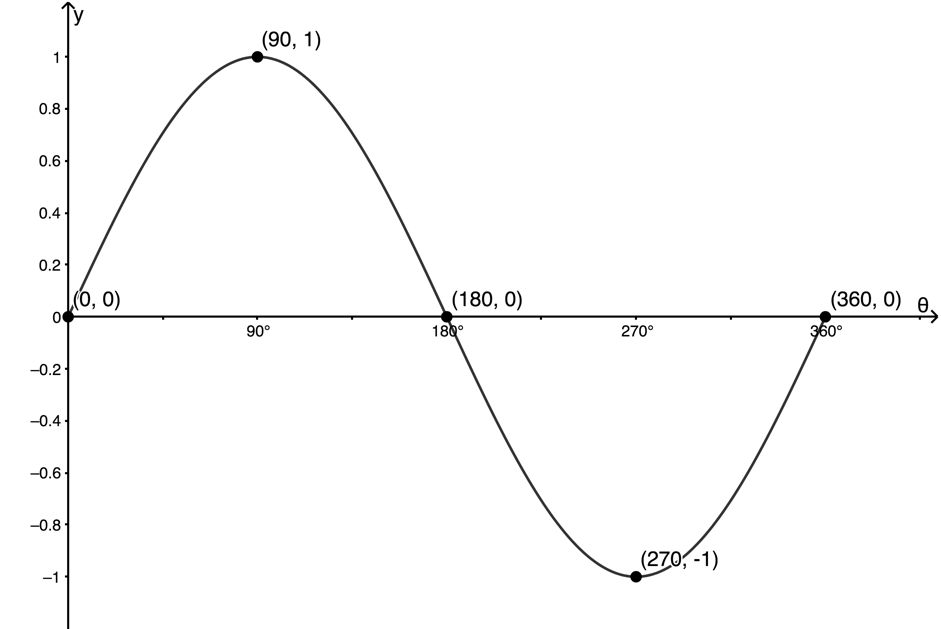Figure 6: Graph of $\scriptsize f(\theta )=\sin \theta$ for $\scriptsize {{0}^{{}^\circ }}\le \theta \le {{360}^{{}^\circ }}$
1. This is what you should notice about the graph.
1. The maximum value the graph reaches is $\scriptsize 1$. Its minimum value is $\scriptsize -1$.
2. The graph has a maximum turning point at $\scriptsize ({{90}^{{}^\circ }},1)$ and a minimum turning point at $\scriptsize ({{270}^{{}^\circ }},-1)$.
3. If we continue past $\scriptsize {{360}^{{}^\circ }}$ or $\scriptsize {{0}^{{}^\circ }}$ the graph will repeat itself. This is because if we keep measuring angles on our unit circle, we will keep generating the same function values. An angle of $\scriptsize {{390}^{{}^\circ }}$ will give a triangle on the unit circle exactly the same as $\scriptsize {{30}^{{}^\circ }}$. If we measured in the other direction, an angle of $\scriptsize -{{30}^{{}^\circ }}$ for example, it would be the same as an angle of $\scriptsize {{330}^{{}^\circ }}$.
4. There are no values that $\scriptsize \theta$ cannot take. Therefore, the domain of the function is $\scriptsize \{\theta \ |\ \theta \in \mathbb{R}\}$. The range of the function is all values between $\scriptsize -1$ and $\scriptsize 1$. Therefore the range is $\scriptsize \{f(\theta )\ |\ f(\theta )\in \mathbb{R}\text{, -1}\le y\le \text{1 }\!\!\}\!\!\text{ }$.

Part B

Table 2 shows the completed table of values of the length of the side adjacent to the angle in each case.

 $\scriptsize \theta$ $\scriptsize {{30}^{{}^\circ }}$ $\scriptsize {{45}^{{}^\circ }}$ $\scriptsize {{60}^{{}^\circ }}$ $\scriptsize {{90}^{{}^\circ }}$ $\scriptsize {{120}^{{}^\circ }}$ $\scriptsize {{135}^{{}^\circ }}$ $\scriptsize {{150}^{{}^\circ }}$ $\scriptsize x$ $\scriptsize 8.66$ $\scriptsize 7.07$ $\scriptsize 5$ $\scriptsize 0$ $\scriptsize 8.66$ $\scriptsize 7.07$ $\scriptsize 8.66$ $\scriptsize \displaystyle \frac{x}{r}$ $\scriptsize 0.866$ $\scriptsize 0.707$ $\scriptsize 0.5$ $\scriptsize 0$ $\scriptsize -0.866$ $\scriptsize -0.707$ $\scriptsize -0.866$
 $\scriptsize \theta$ $\scriptsize {{180}^{{}^\circ }}$ $\scriptsize {{210}^{{}^\circ }}$ $\scriptsize {{240}^{{}^\circ }}$ $\scriptsize {{270}^{{}^\circ }}$ $\scriptsize {{300}^{{}^\circ }}$ $\scriptsize {{330}^{{}^\circ }}$ $\scriptsize {{360}^{{}^\circ }}$ $\scriptsize x$ $\scriptsize -10$ $\scriptsize -8.66$ $\scriptsize 5$ $\scriptsize 0$ $\scriptsize 5$ $\scriptsize -8.66$ $\scriptsize 10$ $\scriptsize \displaystyle \frac{x}{r}$ $\scriptsize -1$ $\scriptsize -0.866$ $\scriptsize -0.5$ $\scriptsize 0$ $\scriptsize 0.5$ $\scriptsize 0.866$ $\scriptsize 1$

When you plot the coordinates, formed by the values of $\scriptsize \theta$ and $\scriptsize \displaystyle \frac{x}{r}$, you get the graph shown in Figure 7.Figure 7: Graph of $\scriptsize f(\theta )=\cos \theta$ for $\scriptsize {{0}^{{}^\circ }}\le \theta \le {{360}^{{}^\circ }}$
1. This is what you should notice about the graph.
1. The maximum value the graph reaches is $\scriptsize 1$. Its minimum value is $\scriptsize -1$.
2. The graph has maximum turning points at $\scriptsize ({{0}^{{}^\circ }},1)$ and $\scriptsize ({{360}^{{}^\circ }},1)$, and a minimum turning point at $\scriptsize ({{180}^{{}^\circ }},-1)$.
3. If we continue past $\scriptsize {{360}^{{}^\circ }}$ or $\scriptsize {{0}^{{}^\circ }}$ the graph will repeat itself. This is because if we keep measuring angles on our unit circle, we will keep generating the same function values.
4. There are no values that $\scriptsize \theta$ cannot take. Therefore, the domain of the function is $\scriptsize \{\theta \ |\ \theta \in \mathbb{R}\}$. The range of the function is all values between $\scriptsize -1$ and $\scriptsize 1$. Therefore the range is $\scriptsize \{f(\theta )\ |\ f(\theta )\in \mathbb{R}\text{, -1}\le y\le \text{1 }\!\!\}\!\!\text{ }$.

Part C

1. Th sine function interactive simulation constructed the graph of the function $\scriptsize f(\theta )=\sin \theta$ for $\scriptsize {{0}^{{}^\circ }}\le \theta \le {{360}^{{}^\circ }}$.
2. Th cosine function interactive simulation constructed the graph of the function $\scriptsize f(\theta )=\cos \theta$ $\scriptsize {{0}^{{}^\circ }}\le \theta \le {{360}^{{}^\circ }}$.

## Amplitude and period

In Activity 5.1 we discovered the general shape of the functions of $\scriptsize y=\sin x$ and $\scriptsize y=\cos x$. We also saw that the graphs have maximum values of $\scriptsize 1$ (a maximum turning point) and minimum values of $\scriptsize -1$ (a minimum turning point) and that they ‘bounce’ between these limits. This is represented by the range $\scriptsize \{f(x)\ |\ f(x)\in \mathbb{R}\text{,}-1\le y\le 1\}$.

We say that each of these functions has an amplitude of $\scriptsize 1$. Amplitude is a measure of the maximum distance of the graph from the ‘middle’ or ‘point of rest’ of the graph. In this case, the point of rest is the x-axis.

Look at Figure 8 which shows the graphs of $\scriptsize y=\sin x$ and $\scriptsize y=\cos x$ for $\scriptsize -{{720}^{{}^\circ }}\le x\le {{720}^{{}^\circ }}$. Here we can clearly see how the graphs repeat themselves again and again. How many degrees does it take for each graph to repeat itself?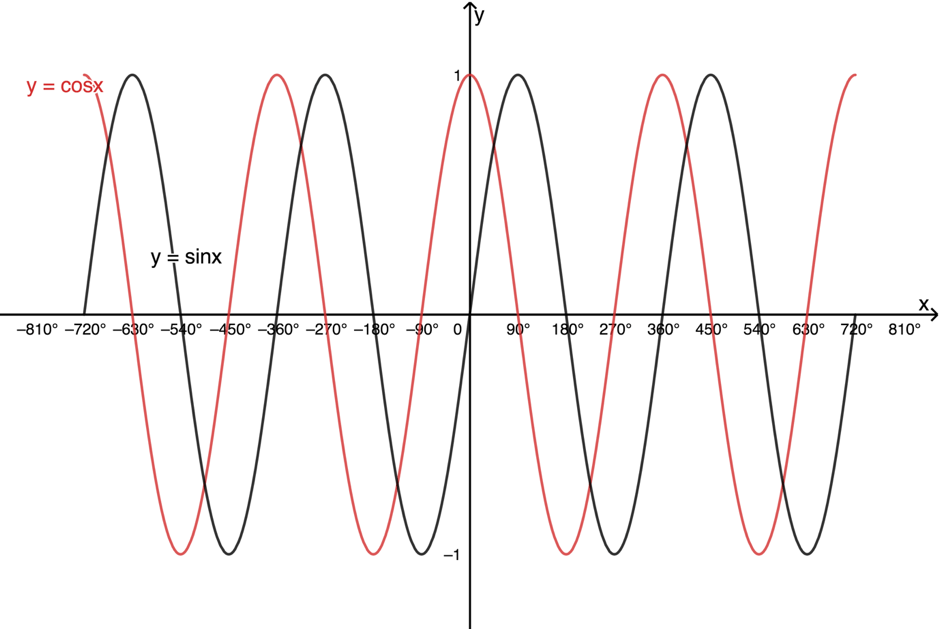Figure 8: Graphs of $\scriptsize y=\sin x$ and $\scriptsize y=\cos x$ for $\scriptsize -{{720}^{{}^\circ }}\le x\le {{720}^{{}^\circ }}$

If you look at Figure 8, you can see that each graph repeats itself every $\scriptsize {{360}^{{}^\circ }}$. We say that each graph has a period of $\scriptsize {{360}^{{}^\circ }}$.

Can you also see that both functions are exactly the same shape? The graph of $\scriptsize y=\cos x$ is really just the graph of $\scriptsize y=\sin x$ shifted $\scriptsize {{90}^{{}^\circ }}$ to the left. You will see in Level 3 why this is the case.

# The effects of a and q on the graphs of y=a.sin x+q and y=a.cos x+q

Before working through Activity 5.2, what do you think the effect of changing the value of $\scriptsize q$ will be on the functions of $\scriptsize y=\sin x+q$ and $\scriptsize y=\cos x+q$? Think about what changing $\scriptsize q$ does to other functions we have studied.

## The effect of $\scriptsize \large q$ on the graphs of $\scriptsize \large y=a\sin x+q$ and $\scriptsize \large y=a\cos x+q$

Work through this activity to understand the effect of q on the sine and cosine functions.### Activity 5.2: Investigate the effect of q on the sine and cosine functions

Time required: 15 minutes

What you need:

• an internet connection

What to do:

When you have an internet connection, visit this interactive simulation.Here you will find graphs of $\scriptsize f(x)=\sin x+q$ and $\scriptsize g(x)=\cos x+q$ with a slider to change the value of $\scriptsize q$. Change the value of $\scriptsize q$ and see the effect on each graph. You can show and hide each function by clicking the coloured circle next to it.

1. What effect does changing $\scriptsize q$ have of each function?
2. Does changing $\scriptsize q$ change the maxima and minima of the functions? If so, how?
3. Does changing $\scriptsize q$ affect the amplitude of the functions?
4. Does changing $\scriptsize q$ affect the period of the functions?

What did you find?

1. By changing the value of $\scriptsize q$ the functions are moved vertically up and down. Increasing $\scriptsize q$, shifts the functions up by $\scriptsize q$ units. Decreasing $\scriptsize q$ shifts the functions down by $\scriptsize q$ units.
2. Changing $\scriptsize q$ does change the maximum and minimum turning points of the functions. The normal maxima and minima of the functions are $\scriptsize 1$ and $\scriptsize -1$. The new values are $\scriptsize 1+q$ and $\scriptsize -1+q$.
3. The amplitude of the functions does not change. The distance from the middle of the graph to the maximum or minimum turning point is still $\scriptsize 1$. However, the position of the ‘middle’ of the graphs does change. This is now the straight line $\scriptsize y=q$.
4. Both graphs have the same period of $\scriptsize {{360}^{{}^\circ }}$.### Example 5.1

Given $\scriptsize y=\sin x-1$.

1. What is the period of the function?
2. What is the amplitude of the function?
3. At what y-coordinates will the maximum and minimum turning points be?
4. State the domain and range.
5. What are the intercepts with the axes?
6. Make a neat sketch of the function for $\scriptsize {{0}^{{}^\circ }}\le x\le {{360}^{{}^\circ }}$.

Solutions

We need to first consider what the basic sine function looks like (see Figure 9).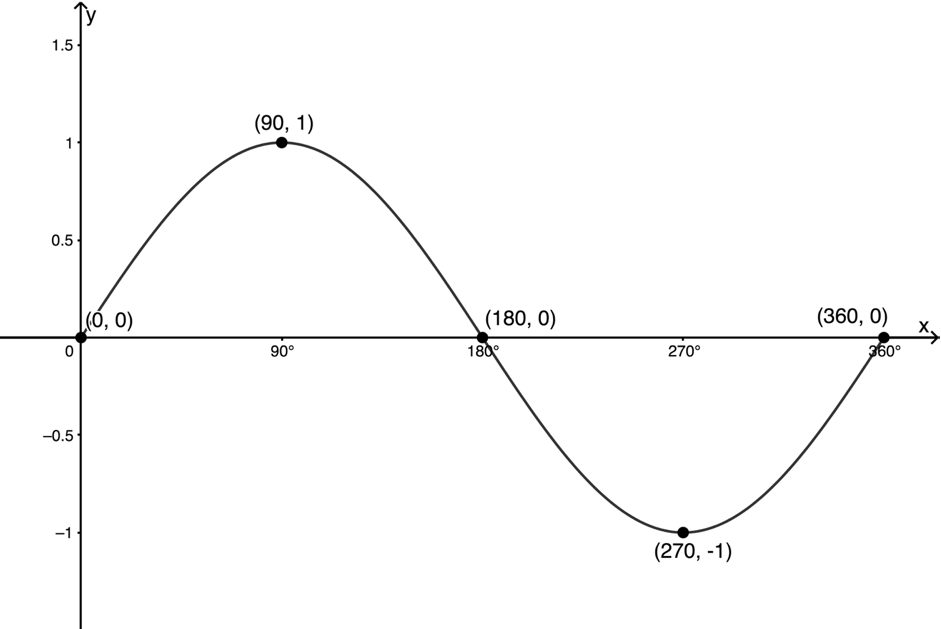Figure 9: Graph of $\scriptsize y=\sin x$ for $\scriptsize {{0}^{{}^\circ }}\le x\le \text{36}{{\text{0}}^{{}^\circ }}$

We can see that the graph passes through the origin to a maximum at $\scriptsize ({{90}^{{}^\circ }},1)$, back through the x-axis at $\scriptsize ({{180}^{{}^\circ }},0)$ to a minimum at $\scriptsize ({{270}^{{}^\circ }},-1)$ and then back to the x-axis at $\scriptsize ({{360}^{{}^\circ }},0)$. The graph has a period of $\scriptsize {{360}^{{}^\circ }}$ and an amplitude of $\scriptsize 1$. The ‘middle’ of the graph is the x-axis (the line $\scriptsize y=0$).

The function we need to sketch is $\scriptsize y=\sin x-1$. Here $\scriptsize q=-1$ and the whole graph in Figure 9 will be moved one unit down.

1. The period of the function will remain unchanged at $\scriptsize {{360}^{{}^\circ }}$.
2. The amplitude of the function will remain unchanged at $\scriptsize 1$. However, the ‘middle’ of the graph will now be the line $\scriptsize y=-1$.
3. Because the whole graph has been moved down one unit, the maximum turning points will have y-coordinates of $\scriptsize 1-1=0$ and the minimum turning points will have y-coordinates of $\scriptsize -1-1=-2$.
4. Domain: $\scriptsize \{x\ |\ x\in \mathbb{R}\}$
Range: $\scriptsize \{y\ |\ y\in \mathbb{R},-2\le y\le 0\}$
5. We find the x- and y-intercepts by letting $\scriptsize y=0$ and $\scriptsize x=0$.
x-intercept (let $\scriptsize y=0$):
\scriptsize \begin{align*}0 & =\sin x-1\\\therefore \sin x & =1\\\therefore x & ={{90}^{{}^\circ }}\end{align*}
The point $\scriptsize ({{90}^{{}^\circ }},0)$ will be the x-intercept.
.
y-intercept (let $\scriptsize x=0$):
\scriptsize \begin{align*}y & =\sin {{0}^{{}^\circ }}-1\\\therefore y & =0-1=-1\end{align*}
The point $\scriptsize (0,-1)$ will be the y-intercept.
6. The graph of $\scriptsize y=\sin x-1$ is shown in Figure 10. The light dotted line represents the ‘middle’ of the function and the dark dotted line the function $\scriptsize y=\sin x$.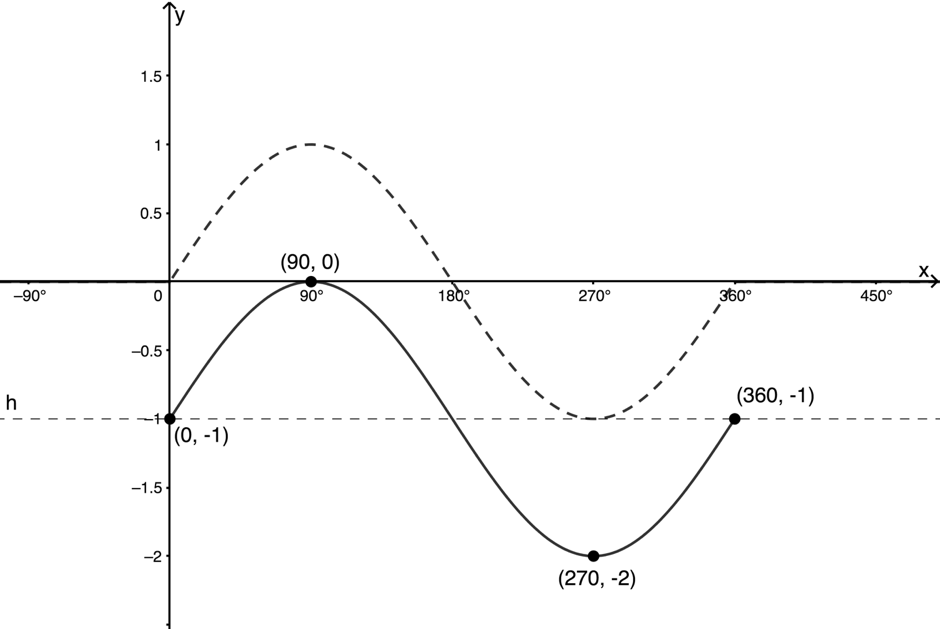Figure 10: Graph of $\scriptsize y=\sin x-1$ for $\scriptsize {{0}^{{}^\circ }}\le x\le \text{36}{{\text{0}}^{{}^\circ }}$

Note: You could also have sketched the function using a table of values or your calculator. When using a table of values, it is often only necessary to use these angles: $\scriptsize {{0}^{{}^\circ }};{{90}^{{}^\circ }};{{180}^{{}^\circ }};{{270}^{{}^\circ }};{{360}^{{}^\circ }}$.

### Note

In Example 5.1 it was necessary to calculate $\scriptsize x$ in $\scriptsize \sin x=1$. You can do this on your calculator by using the inverse sine button, usually marked as $\scriptsize {{\sin }^{-1}}$. For this calculation, key in $\scriptsize 1\to \text{2nd func}\to \sin \to \ =$ (on most Sharp calculators) or $\scriptsize \text{SHIFT}\to \sin \to 1\to =$ (on most Casio calculators).

It was also necessary to calculate $\scriptsize y$ in $\scriptsize y=\sin {{0}^{{}^\circ }}-1$. You can do this on your calculator by using the sine button, marked as $\scriptsize \sin$. For this calculation, key in $\scriptsize 0\to \sin \to -1\to \ =$.

You can also use your calculator to generate sets of ordered pairs for any function. When you have an internet connection, watch the video called “CASIO FX 991ES PLUS – Calculator skills – plotting a graph” to learn how to do this.

CASIO FX 991ES PLUS – Calculator skills – plotting a graph (Duration: 2.59)### Exercise 5.1

Make a neat sketch of the function $\scriptsize f(x)=\sin x+1$ for the interval $\scriptsize {{0}^{{}^\circ }}\le x\le \text{36}{{\text{0}}^{{}^\circ }}$ showing the intercepts with the axes and turning points.

Make a neat sketch of the function $\scriptsize g(\theta )=\cos \theta -3$ for the interval $\scriptsize {{0}^{{}^\circ }}\le \theta \le {{540}^{{}^\circ }}$showing the intercepts with the axes and turning points.

The full solutions are at the end of the unit.

We have established that the effect of $\scriptsize q$ on $\scriptsize y=\sin x+q$ and $\scriptsize y=\cos x+q$ is to move the graph vertically up or down by $\scriptsize q$ units. There is no change in the overall shape of the graph.

## The effect of $\scriptsize \large a$ on $\scriptsize \large y=\sin x+q$ and $\scriptsize \large y=\cos x+q$

Based on your knowledge of the effect of $\scriptsize a$ on the other functions we have studied, what do you think the effect of $\scriptsize a$ will be on $\scriptsize y=a\sin x+q$ and $\scriptsize y=a\cos x+q$?

Consider the following table of values (Table 3) for the function $\scriptsize y=\sin x$.

 $\scriptsize \theta$ $\scriptsize {{0}^{{}^\circ }}$ $\scriptsize {{90}^{{}^\circ }}$ $\scriptsize {{180}^{{}^\circ }}$ $\scriptsize {{270}^{{}^\circ }}$ $\scriptsize {{360}^{{}^\circ }}$ $\scriptsize f(x)=\sin x$ $\scriptsize 0$ $\scriptsize 1$ $\scriptsize 0$ $\scriptsize -1$ $\scriptsize 0$

What values will go into the second row if instead we calculated the values for $\scriptsize g(x)=2\sin x$? What values would go into the second row if we calculated the values for $\scriptsize h(x)=-3\sin x$. Before you read on, make a copy of Table 4 on a piece of paper and try to complete it on your own.

 $\scriptsize \theta$ $\scriptsize {{0}^{{}^\circ }}$ $\scriptsize {{90}^{{}^\circ }}$ $\scriptsize {{180}^{{}^\circ }}$ $\scriptsize {{270}^{{}^\circ }}$ $\scriptsize {{360}^{{}^\circ }}$ $\scriptsize f(x)=\sin x$ $\scriptsize 0$ $\scriptsize 1$ $\scriptsize 0$ $\scriptsize -1$ $\scriptsize 0$ $\scriptsize g(x)=2\sin x$ $\scriptsize h(x)=-3\sin x$

It should be clear that for $\scriptsize g(x)$ we simply double the values obtained for $\scriptsize f(x)$, and that for $\scriptsize h(x)$ we multiply each of these values by $\scriptsize -3$ (see Table 5).

 $\scriptsize \theta$ $\scriptsize {{0}^{{}^\circ }}$ $\scriptsize {{90}^{{}^\circ }}$ $\scriptsize {{180}^{{}^\circ }}$ $\scriptsize {{270}^{{}^\circ }}$ $\scriptsize {{360}^{{}^\circ }}$ $\scriptsize f(x)=\sin x$ $\scriptsize 0$ $\scriptsize 1$ $\scriptsize 0$ $\scriptsize -1$ $\scriptsize 0$ $\scriptsize g(x)=2\sin x$ $\scriptsize 0$ $\scriptsize 2$ $\scriptsize 0$ $\scriptsize -2$ $\scriptsize 0$ $\scriptsize h(x)=-3\sin x$ $\scriptsize 0$ $\scriptsize -3$ $\scriptsize 0$ $\scriptsize 3$ $\scriptsize 0$

This means that the y-coordinate of every point on $\scriptsize g(x)=2\sin x$ is going to be double the y-coordinate of every point on $\scriptsize f(x)=\sin x$. The y-coordinate of every point on $\scriptsize h(x)=-3\sin x$ is going to be three times the y-coordinate of every point on $\scriptsize f(x)=\sin x$ and on the opposite side of the x-axis.

Figure 11 shows the graphs of these three functions. From it, we can see how $\scriptsize g(x)=2\sin x$ is the same basic shape as $\scriptsize f(x)=\sin x$, but that the graph has been vertically stretched so that the maxima and minima are now $\scriptsize 2$ and $\scriptsize -2$ instead of $\scriptsize 1$ and $\scriptsize -1$. This means that the range of $\scriptsize g(x)=2\sin x$ has been extended to be $\scriptsize \{g(x)\ |\ g(x)\in \mathbb{R},-2\le g(x)\le 2\}$. The amplitude of $\scriptsize g(x)$ is $\scriptsize 2$.

The domain and period of $\scriptsize g(x)$ are the same as for $\scriptsize f(x)$.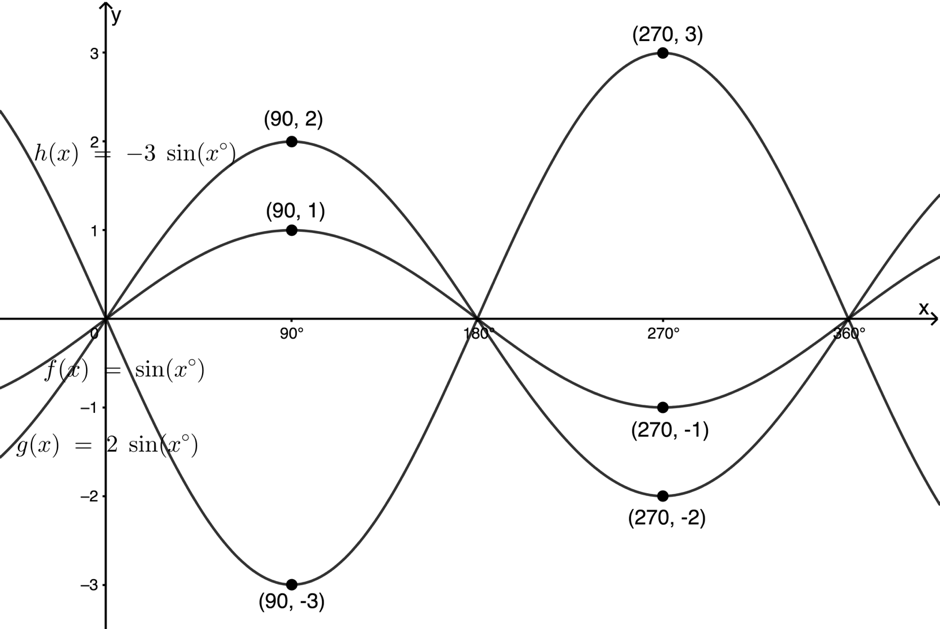Figure 11: Graphs of $\scriptsize f(x)=\sin x$, $\scriptsize g(x)=2\sin x$ and $\scriptsize h(x)=-3\sin x$

We can also see how $\scriptsize h(x)=-3\sin x$ is the same basic shape as $\scriptsize f(x)$, but that the graph has been vertically stretched so that the maxima and minima are now $\scriptsize 3$ and $\scriptsize -3$ instead of $\scriptsize 1$ and $\scriptsize -1$. Because $\scriptsize a \lt 0$ it also means that the graph has been flipped over the x-axis. What was a maximum value is now a minimum.

This means that the range of $\scriptsize h(x)=-3\sin x$ has been extended to $\scriptsize \{h(x)\ |\ h(x)\in \mathbb{R},-3\le h(x)\le 3\}$. The amplitude of $\scriptsize h(x)$ is $\scriptsize 3$.

The domain and period of $\scriptsize h(x)$ are the same as for $\scriptsize f(x)$.

Naturally, the effect of $\scriptsize a$ on the function $\scriptsize y=a\cos x$ will be the same. The graph will be stretched vertically and the new maxima and minima will be multiplies of $\scriptsize a$. Also, if $\scriptsize a \lt 0$, the graph will be flipped over the x-axis and what was a maximum value will become a minimum value.

Before you look at the next example, visit this interactive simulation.Here you will find graphs of $\scriptsize f(x)=a\sin x+q$ and $\scriptsize g(x)=a\cos x+q$ with sliders to change the values of $\scriptsize a$ and $\scriptsize q$. Change the values to see the effect on each graph. You can show and hide each function by clicking the coloured circle next to it.### Example 5.2

Given $\scriptsize s(x)=3\cos x-1$.

1. What is the period of the function?
2. What is the amplitude of the function?
3. Find the y-coordinates of the maximum and minimum turning points?
4. State the domain and range.
5. What are the intercepts with the axes (to one decimal place)?
6. Make a neat sketch of the function for $\scriptsize {{0}^{{}^\circ }}\le x\le {{360}^{{}^\circ }}$, marking the turning points and intercepts.

Solutions

1. The period is $\scriptsize {{360}^{{}^\circ }}$.
2. The of $\scriptsize a$ is $\scriptsize 3$ therefore, we can say the amplitude of the function is $\scriptsize 3$.
3. $\scriptsize q=-1$. Therefore, the whole graph will be shifted one unit down. The ‘middle’ of the graph will be the line $\scriptsize y=-1$. This means that the maxima will be at $\scriptsize 3-1=2$ and the minima will be at $\scriptsize -3-1=-4$.
4. Domain: $\scriptsize x\in \mathbb{R}$
Range: $\scriptsize y\in [-4,2]$
Note: These shortened representations of the domain and range are perfectly acceptable. The range has been represented in interval notation where the square brackets indicate that the end values are included. Interval notation assumes that all real values between the end values are included.
5. x-intercept (let $\scriptsize y=0$):
\scriptsize \begin{align*}0 & =3\cos x-1\\\therefore 3\cos x & =1\\\therefore \cos x & =\displaystyle \frac{1}{3}\\\therefore x & ={{70.5}^{{}^\circ }}\end{align*}
The point $\scriptsize ({{70.5}^{{}^\circ }},0)$ will be the x-intercept. y-intercept (let $\scriptsize x=0$):
\scriptsize \begin{align*}y & =3\cos {{0}^{{}^\circ }}-1\\\therefore y & =3-1=2\end{align*}
The point $\scriptsize (0,2)$ will be the y-intercept.

Figure 12 shows a sketch of the function.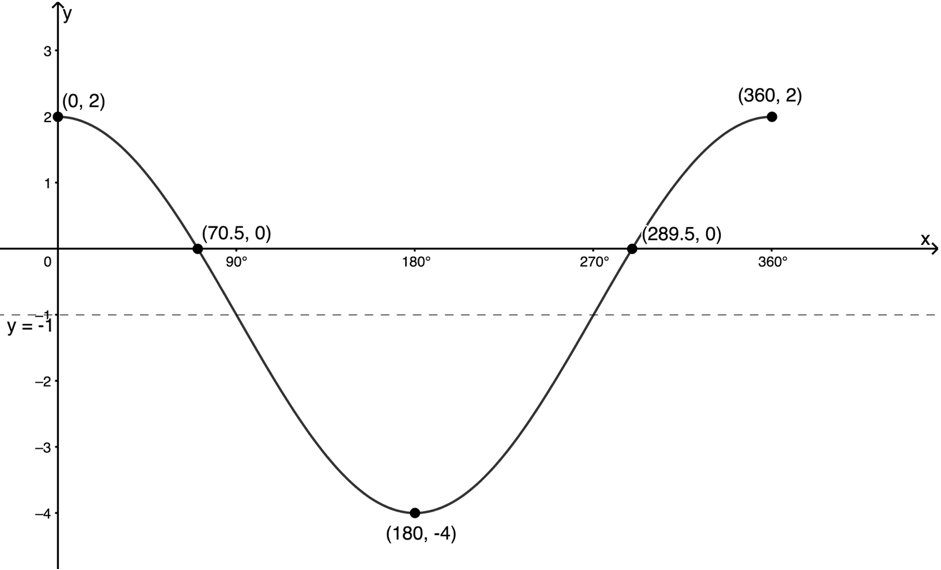Figure 12: Graph of $\scriptsize s(x)=3\cos x-1$ for $\scriptsize {{0}^{{}^\circ }}\le x\le {{360}^{{}^\circ }}$

### Note

You will notice in Figure 12 that a second x-intercept of $\scriptsize ({{289.5}^{{}^\circ }},0)$ is shown that was not calculated in the example. You will learn how to calculate the general solution for trigonometric equations in Level 3.### Exercise 5.2

1. Sketch $\scriptsize y=-2\sin x-2$ for $\scriptsize x\in [{{0}^{{}^\circ }},{{180}^{{}^\circ }}]$ showing all the intercepts with the axes and the maxima and minima.
2. Sketch $\scriptsize y=-\displaystyle \frac{1}{2}\cos x+1$ for $\scriptsize x\in [{{0}^{{}^\circ }},{{180}^{{}^\circ }}]$ showing all the intercepts with the axes and the maxima and minima.

The full solutions are at the end of the unit.

Figures 13 and 14 show a summary of what we know about the effects of $\scriptsize a$ and $\scriptsize q$ on the functions $\scriptsize y=a\sin x+q$ and $\scriptsize y=a\cos x+q$.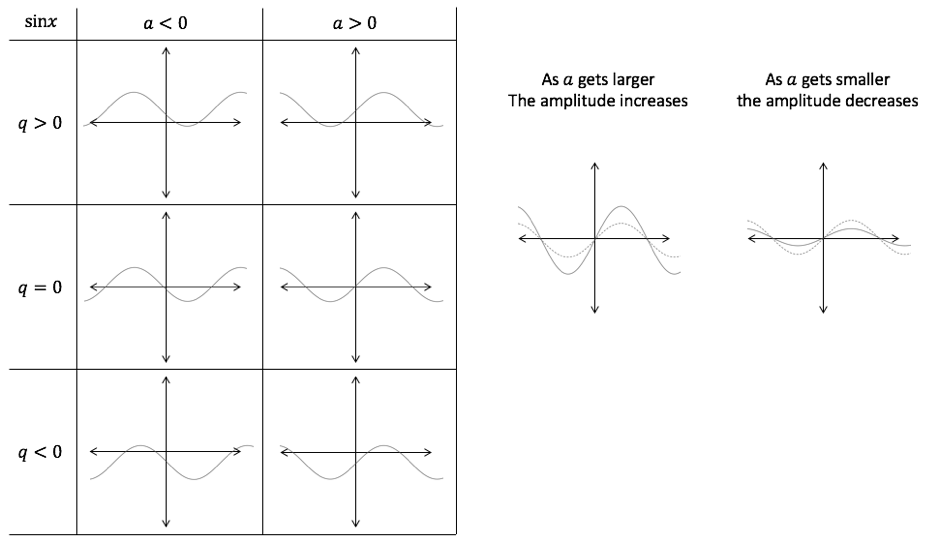Figure 13: Effects of $\scriptsize a$ and $\scriptsize q$ on the function $\scriptsize y=a\sin x+q$

For $\scriptsize y=a\sin x+q$
Period: $\scriptsize {{360}^{{}^\circ }}$
Amplitude: $\scriptsize |a|$ (the of $\scriptsize a$)
Domain: $\scriptsize x\in \mathbb{R}$
Range: $\scriptsize y\in [a+q,-a+q]$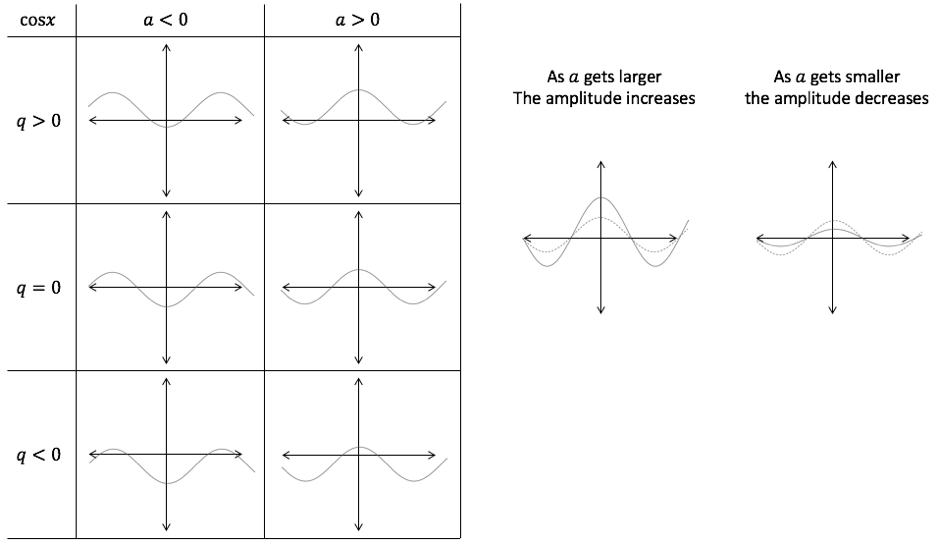Figure 14: Effects of $\scriptsize a$ and $\scriptsize q$ on the function $\scriptsize y=a\cos x+q$

For $\scriptsize y=a\cos x+q$
Period: $\scriptsize {{360}^{{}^\circ }}$
Amplitude: $\scriptsize |a|$ (the of $\scriptsize a$)
Domain: $\scriptsize x\in \mathbb{R}$
Range: $\scriptsize y\in [a+q,-a+q]$

# The tangent function

We know that the tangent function is the ratio of the length of the side opposite the angle to the length of the side adjacent to the angle. In other words $\scriptsize \tan \theta =\displaystyle \frac{y}{x}$. But if we consider the point $\scriptsize (x,y)$ as the point at the end of a radius drawn at an angle of $\scriptsize \theta$ from the positive x-axis at the origin in the unit circle, we can see that the tangent function is actually the gradient of this radius (see Figure 15).

## The basic tangent function

Let’s think about how the gradient or slope of the radius in Figure 15 changes as we increase the angle from $\scriptsize {{0}^{{}^\circ }}$ to $\scriptsize {{360}^{{}^\circ }}$. If you have an Internet connection, visit the Interactive tangent function simulation.Drag the green slider to change the angle. Notice how the gradient of the radius in the unit circle corresponds to the function value.

When the angle the radius of the unit circle makes with the x-axis is $\scriptsize {{0}^{{}^\circ }}$, the slope of the radius is also zero and, therefore, $\scriptsize \tan {{0}^{{}^\circ }}=0$ (see Figure 16). As the angle increases, so does the slope and so does the value of $\scriptsize \tan \theta$. When $\scriptsize \theta ={{45}^{{}^\circ }}$, the slope of the radius is $\scriptsize 1$ and so $\scriptsize \tan {{45}^{{}^\circ }}=1$ (see Figure 17). However, as $\scriptsize \theta$ approaches $\scriptsize {{90}^{{}^\circ }}$, the slope of the radius increases and, as it gets more and more vertical, it approaches infinity. Therefore, the graph of the tangent function has an asymptote at $\scriptsize \theta ={{90}^{{}^\circ }}$ because $\scriptsize \tan {{90}^{{}^\circ }}$ is undefined (see Figure 18).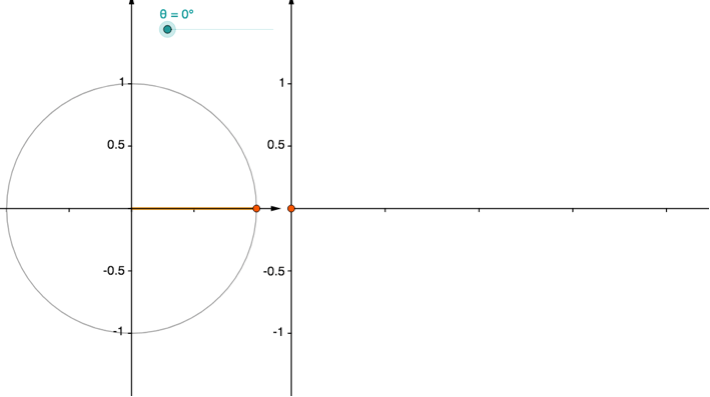Figure 16: Tangent function value when $\scriptsize \theta ={{0}^{{}^\circ }}$Figure 17: Tangent function value when $\scriptsize \theta ={{45}^{{}^\circ }}$Figure 18: Tangent function value when $\scriptsize \theta ={{90}^{{}^\circ }}$

On the other side of $\scriptsize \theta ={{90}^{{}^\circ }}$, the gradient of the line suddenly switches to being very large negative but gradually decreases until it is $\scriptsize -1$ when $\scriptsize \theta ={{135}^{{}^\circ }}$ (see Figure 19). When $\scriptsize \theta ={{180}^{{}^\circ }}$, the slope is again zero and so $\scriptsize \tan {{180}^{{}^\circ }}=0$ (see Figure 20).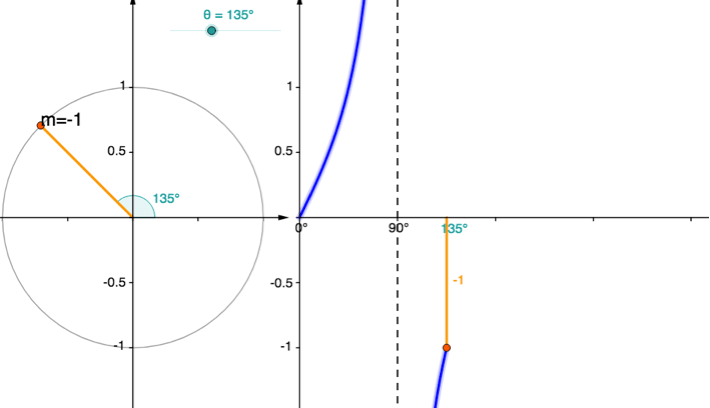Figure 19: Tangent function value when $\scriptsize \theta ={{135}^{{}^\circ }}$Figure 20: Tangent function value when $\scriptsize \theta ={{180}^{{}^\circ }}$

As $\scriptsize \theta$ continues to increase, the gradient becomes positive again and increases to a value of $\scriptsize 1$ when $\scriptsize \theta ={{225}^{{}^\circ }}$ (see Figure 21). As $\scriptsize \theta$ approaches $\scriptsize {{270}^{{}^\circ }}$, the slope again approaches infinity and we have another asymptote at $\scriptsize {{270}^{{}^\circ }}$ (see Figure 22). After this, the slope again switches to being negative and $\scriptsize \tan {{315}^{{}^\circ }}=-1$ and $\scriptsize \tan {{360}^{{}^\circ }}=0$ (see Figure 23).Figure 21: Tangent function value when $\scriptsize \theta ={{225}^{{}^\circ }}$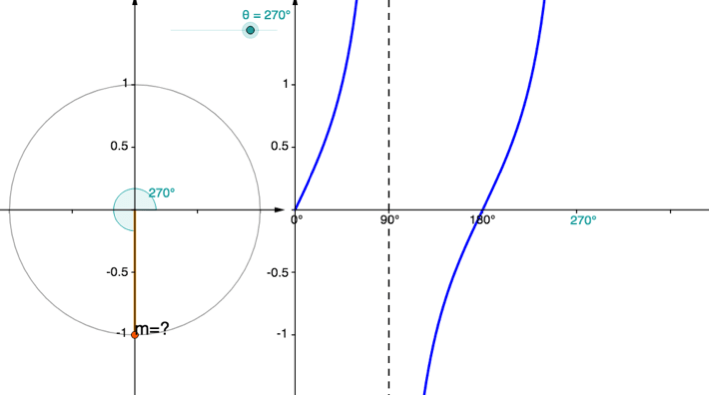Figure 22: Tangent function value when $\scriptsize \theta ={{270}^{{}^\circ }}$Figure 23: Tangent function value when $\scriptsize \theta ={{360}^{{}^\circ }}$

### Domain, range, amplitude and period

In Figure 24 we can see the graph of the function $\scriptsize y=\tan x$ for $\scriptsize {{0}^{{}^\circ }}\le \theta \le {{360}^{{}^\circ }}$. The period of the function is $\scriptsize {{180}^{{}^\circ }}$. It repeats itself every $\scriptsize {{180}^{{}^\circ }}$.

The graph in Figure 24 has vertical asymptotes at $\scriptsize \theta ={{90}^{{}^\circ }}$ and $\scriptsize \theta ={{270}^{{}^\circ }}$, the domain of the function is all real values except $\scriptsize {{90}^{{}^\circ }}$ and $\scriptsize {{270}^{{}^\circ }}$ in this interval. We write the domain as $\scriptsize \{\theta \ |\ \theta \in \mathbb{R}\text{,}{{\text{0}}^{{}^\circ }}\le \theta \le {{360}^{{}^\circ }},\theta \ne {{90}^{{}^\circ }};{{270}^{{}^\circ }}\}$. In general, we can write the domain as $\scriptsize \{\theta \ |\ \theta \in \mathbb{R}\text{,}\theta \ne (2k-1){{90}^{{}^\circ }},k\in \mathbb{Z}\}$. This might look long and complicated but all it says is that we have excluded every odd multiple of $\scriptsize {{90}^{{}^\circ }}$.Figure 24: Graph of $\scriptsize y=\tan x$ for $\scriptsize {{0}^{{}^\circ }}\le \theta \le {{360}^{{}^\circ }}$

Because the asymptotes are vertical, they do not impact the values that the function can have, hence the range is $\scriptsize y\in \mathbb{R}$. For this reason, the graph of the tangent function does not have a defined amplitude.

The graph has intercepts with the axes but it also has some other important ‘anchor points’ that help when sketching the graph as we shall see. These are the points $\scriptsize ({{45}^{{}^\circ }},1)$, $\scriptsize ({{135}^{{}^\circ }},-1)$,$\scriptsize ({{225}^{{}^\circ }},1)$ and $\scriptsize ({{315}^{{}^\circ }},-1)$.

# The effects of a and q on the graph of y=a.tan x+q

The effects of $\scriptsize a$ and $\scriptsize q$ on the tangent function are quite similar to those on the sine and cosine functions even if the graph looks very different.

Changing $\scriptsize q$ moves the whole graph vertically up or down by $\scriptsize q$ units. Changing $\scriptsize a$ stretches or squashes the graph vertically. If $\scriptsize a \lt 0$, the graph is flipped over the x-axis.### Activity 5.3: Investigate the effect of a and q on the tangent function

Time required: 15 minutes

What you need:

• an internet connection

What to do:

When you have an internet connection, visit this interactive simulation.Here you will find a graph of $\scriptsize f(x)=a\tan x+q$ with sliders to change the value of $\scriptsize a$ and $\scriptsize q$. Change the value of $\scriptsize q$ only.

1. What affect does changing $\scriptsize q$ have on the function and, in particular, the value of the seven labelled anchor points?
2. Does changing $\scriptsize q$ affect the period of the function?
3. Does changing $\scriptsize q$ affect the asymptotes of the function?

Set $\scriptsize q$ back to zero and change the value of $\scriptsize a$.

1. What affect does increasing $\scriptsize a$ have on the function and in particular the value of the seven labelled anchor points?
2. What affect does $\scriptsize a \lt 0$ have on the function and in particular the value of the seven labelled anchor points?
3. Does changing $\scriptsize a$ affect the period of the function?
4. Does changing $\scriptsize a$ affect the asymptotes of the function?

Now change the values of $\scriptsize a$ and $\scriptsize q$ together.

1. What affect will $\scriptsize a=-2$ and $\scriptsize q=-1$ have on the graph and, in particular, the value of the seven anchor points? Make the changes to see if you are correct.

What did you find?

1. If we increase $\scriptsize q$ the graph moves up by $\scriptsize q$ units. If we decrease $\scriptsize q$, the graph moves down by $\scriptsize q$ units. The y-coordinates of all seven anchor points increase or decrease by $\scriptsize q$ units. The overall shape of the graph stays the same.
2. Changing $\scriptsize q$ does not affect the period of the graph. It remains $\scriptsize {{180}^{{}^\circ }}$.
3. Changing $\scriptsize q$ does not affect the asymptotes. These remain at $\scriptsize {{90}^{{}^\circ }}$ and $\scriptsize {{270}^{{}^\circ }}$. This is in keeping with the fact that the period does not change.
4. If we increase the value of $\scriptsize a$, we stretch the graph out vertically. The intercepts with the x-axis remain as they are but the other four anchor points move further away from the axis by a factor of $\scriptsize a$ units. This makes sense if we think about the fact that every value of $\scriptsize \tan x$ is being multiplied by $\scriptsize a$ to produce the final function value.
5. As soon as $\scriptsize a \lt 0$ the graph flips over the x-axis. The intercepts with the x-axis remain the same but the anchor points that were above the axis move below the axis and vice versa. Now each value of $\scriptsize \tan x$ is being multiplied by a negative number so values that were positive become negative and those that were negative become positive.
6. Changing $\scriptsize a$ does not affect the period of the graph. It remains $\scriptsize {{180}^{{}^\circ }}$.
7. Changing $\scriptsize a$ does not affect the asymptotes. These remain at $\scriptsize {{90}^{{}^\circ }}$ and $\scriptsize {{270}^{{}^\circ }}$. This is in keeping with the fact that the period does not change.
8. If $\scriptsize a=2$, the graph will be stretched vertically and flipped over the x-axis. The x-intercepts will not change but the other anchor points will be two units away from the x-axis but on opposite sides to where they were. The point $\scriptsize ({{45}^{{}^\circ }},1)$, for example, will now be at $\scriptsize ({{45}^{{}^\circ }},-2)$ If $\scriptsize q=-1$ this whole altered graph will move one unit down. This will affect all the anchor points. The point $\scriptsize ({{45}^{{}^\circ }},-2)$, for example, will move to $\scriptsize ({{45}^{{}^\circ }},-3)$.### Example 5.3

Given $\scriptsize t(x)=-2\tan x+2$.

1. State the period of the function.
2. State the asymptotes of the function.
3. What are the intercepts with the axes?
4. State the domain and range of the function.
5. Make a neat sketch of the function for $\scriptsize {{0}^{{}^\circ }}\le x\le {{180}^{{}^\circ }}$ showing all the key points.

Solutions

1. Period is $\scriptsize {{180}^{{}^\circ }}$.
2. Asymptotes are $\scriptsize x={{90}^{{}^\circ }}$ and $\scriptsize x={{270}^{{}^\circ }}$.
3. x-intercepts (let $\scriptsize y=0$):
\scriptsize \begin{align*}0 & =-2\tan x+2\\\therefore 2\tan x & =2\\\therefore \tan x & =1\\\therefore x & ={{45}^{{}^\circ }}\end{align*}
x-intercept is the point $\scriptsize ({{45}^{{}^\circ }},0)$
.
y-intercept (let $\scriptsize x=0$):
\scriptsize \begin{align*}t(x)=-2\tan {{0}^{{}^\circ }}+2\\\therefore t(x)=2\end{align*}
y-intercept is the point $\scriptsize ({{0}^{{}^\circ }},2)$
4. Domain: $\scriptsize \{x\ |\ x\in \mathbb{R},x\ne {{90}^{{}^\circ }};{{270}^{{}^\circ }}\}$
Range: $\scriptsize t(x)\in \mathbb{R}$
5. To make a sketch, we need to think about how the normal anchor points on the tangent graph have been changed. A useful approach is to use a table of these points for at least one period.
 Original point After $\scriptsize a$ After $\scriptsize q$ $\scriptsize ({{0}^{{}^\circ }},0)$ $\scriptsize ({{0}^{{}^\circ }},0)$ $\scriptsize ({{0}^{{}^\circ }},2)$ Note: This is the y-intercept we calculated $\scriptsize ({{45}^{{}^\circ }},1)$ $\scriptsize ({{45}^{{}^\circ }},-2)$ $\scriptsize ({{45}^{{}^\circ }},0)$ Note: This is the x-intercept we calculated $\scriptsize ({{135}^{{}^\circ }},-1)$ $\scriptsize ({{135}^{{}^\circ }},2)$ $\scriptsize ({{135}^{{}^\circ }},4)$ $\scriptsize ({{180}^{{}^\circ }},0)$ $\scriptsize ({{180}^{{}^\circ }},0)$ $\scriptsize ({{180}^{{}^\circ }},2)$

We know that there is an asymptote at $\scriptsize x={{90}^{{}^\circ }}$ and that the graph is flipped over the x-axis so therefore, it approaches negative infinity from the left of the asymptote and positive infinity from the right (the opposite way around to normal). Figure 25 shows the sketch of $\scriptsize t(x)=-2\tan x+2$ for $\scriptsize {{0}^{{}^\circ }}\le x\le {{180}^{{}^\circ }}$.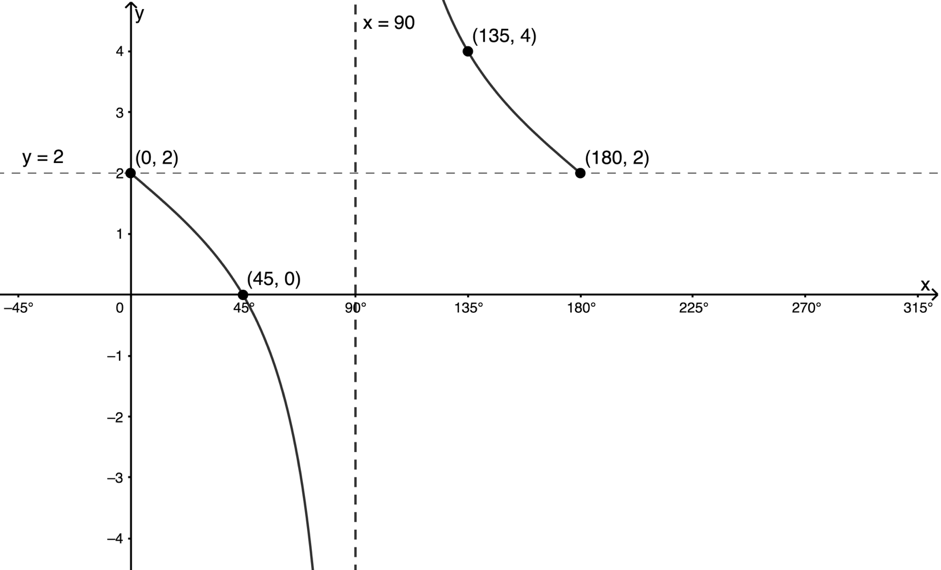Figure 25: Graph of $\scriptsize t(x)=-2\tan x+2$ for $\scriptsize {{0}^{{}^\circ }}\le x\le {{180}^{{}^\circ }}$### Exercise 5.3

Make a neat sketch of $\scriptsize v(x)=3\tan x-1$ for the interval $\scriptsize {{0}^{{}^\circ }}\le x\le {{180}^{{}^\circ }}$.

The full solutions are at the end of the unit.

Figure 26 shows a summary of what we know about the effects of $\scriptsize a$ and $\scriptsize q$ on the function $\scriptsize y=a\tan x+q$.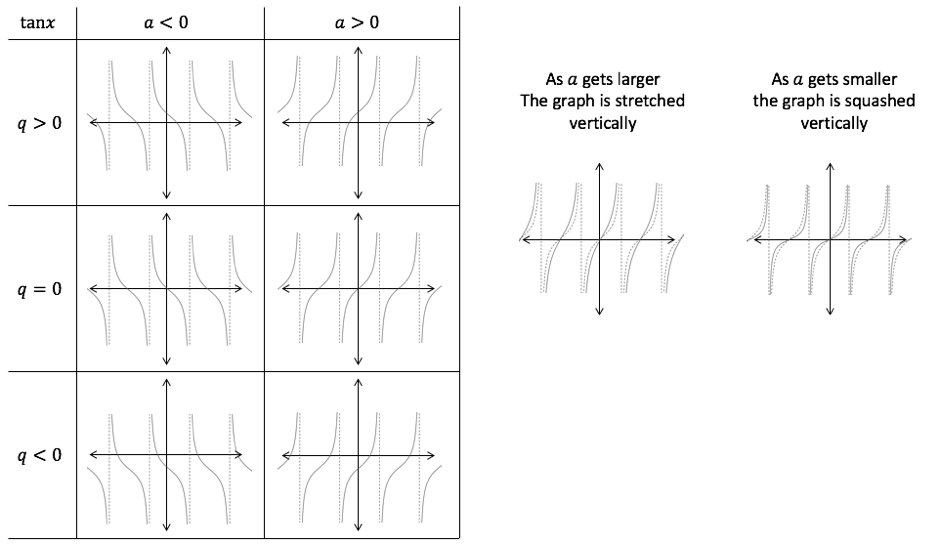Figure 26: Effects of $\scriptsize a$ and $\scriptsize q$ on the function $\scriptsize y=a\tan x+q$

For $\scriptsize y=a\tan x+q$
Period: $\scriptsize {{180}^{{}^\circ }}$
Asymptotes: $\scriptsize (2k-1){{90}^{{}^\circ }},k\in \mathbb{Z}$
Domain: $\scriptsize x\in \mathbb{R},x\ne (2k-1){{90}^{{}^\circ }},k\in \mathbb{Z}$
Range: $\scriptsize y\in \mathbb{R}$

# Finding the equations of trigonometric functions

In this section, we will finding the equations of functions of the form $\scriptsize y=a\sin x+q$, $\scriptsize y=a\cos x+q$ and $\scriptsize y=a\tan x+q$.### Example 5.4

1. Figure 27 shows the graph of the function $\scriptsize f(x)=a\sin x+q$ where $\scriptsize \text{A}({{90}^{{}^\circ }},2)$ and $\scriptsize \text{B}({{180}^{{}^\circ }},-1)$. Find the values of $\scriptsize a$ and $\scriptsize q$.Figure 27: Graph of $\scriptsize f(x)=a\sin x+q$
2. Figure 28 shows the graph of $\scriptsize g(x)=a\cos x+q$ with $\scriptsize \text{C}({{0}^{{}^\circ }},-2.5)$ and $\scriptsize \text{D}({{180}^{{}^\circ }},-1.5)$. Find the values of $\scriptsize a$ and $\scriptsize q$.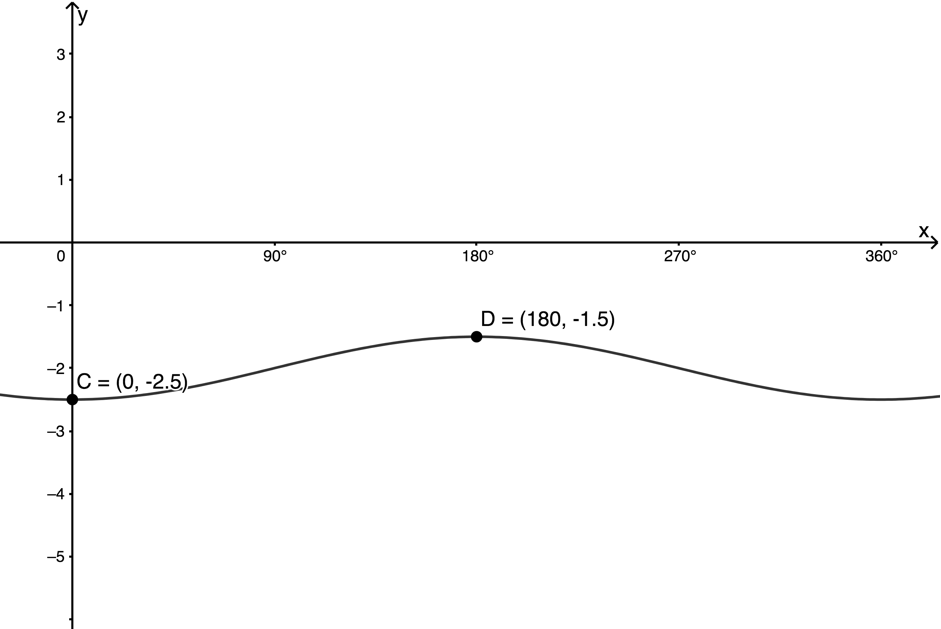Figure 28: Graph of $\scriptsize g(x)=a\cos x+q$
3. Figure 29 shows the graph of $\scriptsize q(x)=a\tan x+q$ with two points shown with coordinates $\scriptsize ({{135}^{{}^\circ }},0)$ and $\scriptsize ({{180}^{{}^\circ }},1.5)$. Find the values of $\scriptsize a$ and $\scriptsize q$.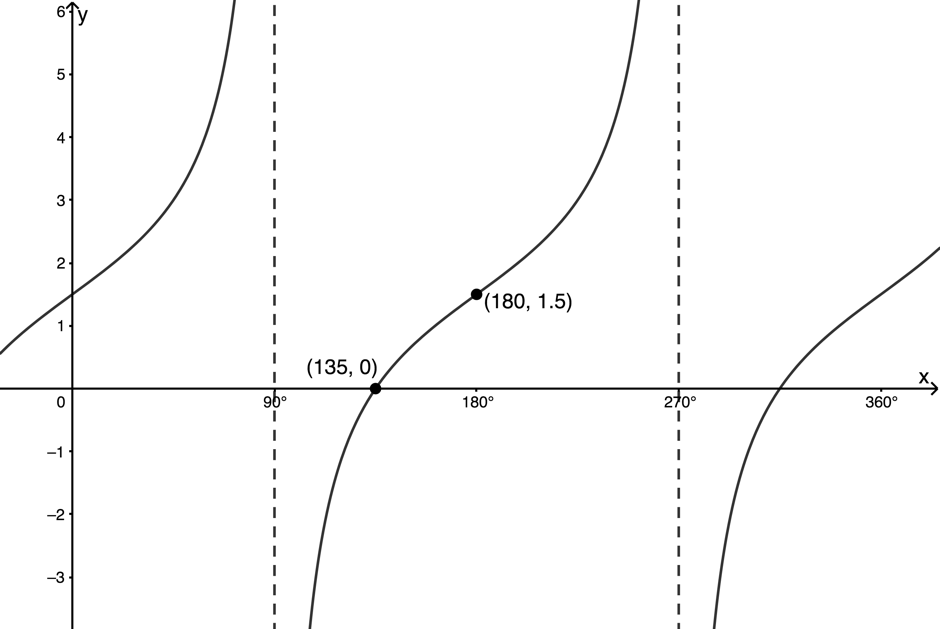Figure 29: $\scriptsize q(x)=a\tan x+q$

Solutions

1. The graph has a y-intercept of $\scriptsize -1$. We know that sine normally has a y-intercept of zero. Therefore, $\scriptsize f(x)$ has been shifted down by one unit. Therefore $\scriptsize q=-1$.
.
If the line $\scriptsize y=-1$ is the new ‘middle’ of the graph, we can see that the amplitude of the graph is now $\scriptsize 3$. Therefore $\scriptsize a=3$.
.
We could also find $\scriptsize a$ algebraically as follows:
$\scriptsize f(x)=a\sin x-1$. Substitute in $\scriptsize \text{A}({{90}^{{}^\circ }},2)$.
\scriptsize \begin{align*} 2 & =a\sin {{90}^{{}^\circ }}-1 \\ \therefore 2 & =a-1\ &&\text{Remember that } \sin90^\circ=1\\ \therefore a & =3 \end{align*}
2. The graph has a y-intercept of $\scriptsize -2.5$. We know that cosine normally has a y-intercept of $\scriptsize 1$. Therefore, it looks like $\scriptsize g(x)$ has been shifted down by $\scriptsize 3.5$ units. However, if that were the case, the new ‘middle’ of the graph would be the line $\scriptsize y=-3.5$ which is not the case. Point $\scriptsize \text{C}$ represents a minimum and point $\scriptsize \text{D}$ a maximum. We can see that the line half way between these points is $\scriptsize y=-2$. This means that $\scriptsize q=-2$.
.
Based on the fact that the new middle of the graph is the line $\scriptsize y=-2$, we can see that the amplitude of the graph is $\scriptsize \displaystyle \frac{1}{2}$. However, cosine normally has a minimum value at $\scriptsize {{180}^{{}^\circ }}$ but this graph has a maximum value. Therefore $\scriptsize a=-\displaystyle \frac{1}{2}$.
.
We could also solve for $\scriptsize a$ algebraically.
$\scriptsize g(x)=a\cos x-2$. Substitute in $\scriptsize \text{A}({{0}^{{}^\circ }},-2.5)$.
\scriptsize \begin{align*}-2.5=a\cos {{0}^{{}^\circ }}-2\\\therefore -2.5=a-2\\\therefore a=-0.5\end{align*}
3. The basic tangent function has an x-intercept at $\scriptsize ({{180}^{{}^\circ }},0)$. This function has this point shifted up by $\scriptsize 1.5$ units. Therefore, $\scriptsize q=1.5$.
.
The shape of the function is the same as the base tangent function. It has not been flipped over the x-axis. Therefore, we know that $\scriptsize a \gt 0$.
.
The anchor point at $\scriptsize ({{135}^{{}^\circ }},-1)$ is now at $\scriptsize ({{135}^{{}^\circ }},0)$. If $\scriptsize a=1$ this point would be at $\scriptsize ({{135}^{{}^\circ }},0.5)$. Thus we know that $\scriptsize a\ne 1$. We can solve for $\scriptsize a$ algebraically. $\scriptsize q(x)=a\tan x+1.5$. Substitute $\scriptsize ({{135}^{{}^\circ }},0)$.
\scriptsize \begin{align*}0=a\tan {{135}^{{}^\circ }}+1.5\\\therefore 0=-a+1.5\\\therefore a=1.5\end{align*}### Exercise 5.4

1. $\scriptsize \text{A}$ lies on $\scriptsize f(x)=\cos x-2$, $\scriptsize \text{B}$ lies on $\scriptsize g(x)=-3\sin x+1$, and$\scriptsize \text{D}$ lies on $\scriptsize j(x)=-2\tan x$.
1. State the coordinates of $\scriptsize \text{A}$, $\scriptsize \text{B}$, and $\scriptsize \text{D}$.
2. State the range of $\scriptsize f(x)$, $\scriptsize g(x)$, and $\scriptsize j(x)$.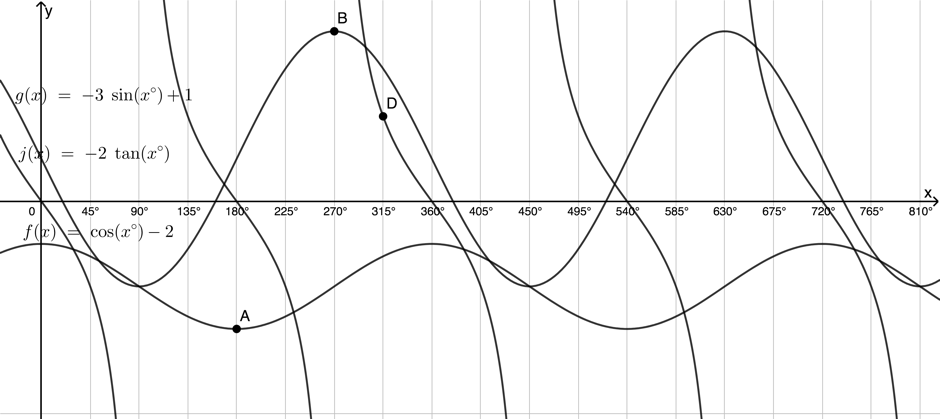Figure 30: Graph of $\scriptsize y=a\cos x+q$
1. Figure 31 shows the graph of $\scriptsize y=a\cos x+q$. Determine the equation of the function.Figure 31: Graph of $\scriptsize y=a\cos x+q$
1. Figure 32 shows a sketch of $\scriptsize r(x)=a\tan x+q$. Determine the values of $\scriptsize a$ and $\scriptsize q$ from the figure.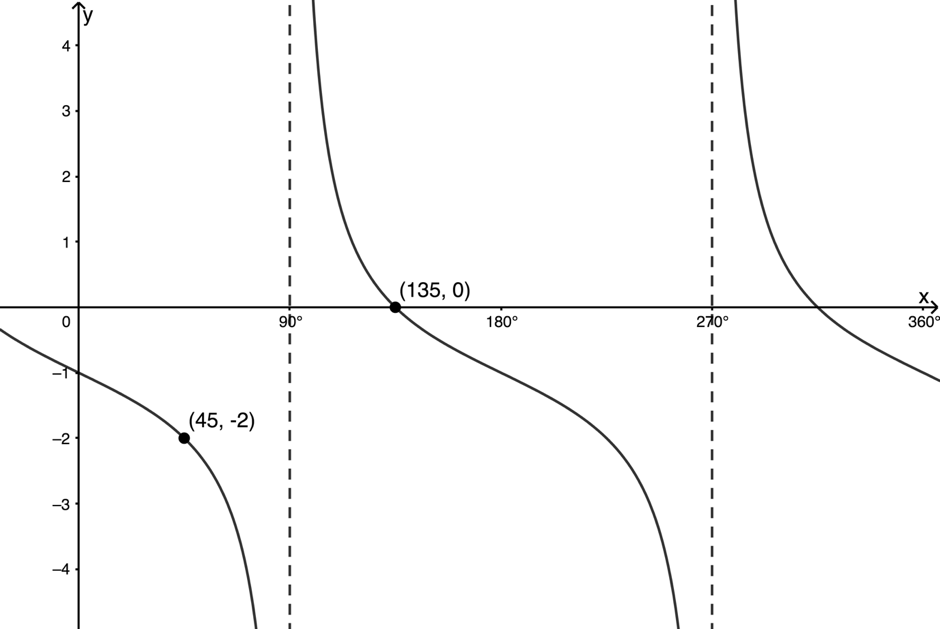Figure 32: Graph of $\scriptsize r(x)=a\tan x+q$

The full solutions are at the end of the unit.

## Summary

In this unit you have learnt the following:

• How to sketch trigonometric functions of the form $\scriptsize y=a\sin x+q$, $\scriptsize y=a\cos x+q$ and $\scriptsize y=a\tan x+q$.
• How to find the equation of graphs of the form $\scriptsize y=a\sin x+q$, $\scriptsize y=a\cos x+q$ and $\scriptsize y=a\tan x+q$.
• The effect of $\scriptsize a$ and $\scriptsize q$ on the shape and position of $\scriptsize y=a\sin x+q$, $\scriptsize y=a\cos x+q$ and $\scriptsize y=a\tan x+q$.
• How to find the asymptotes of functions of the form $\scriptsize y=a\tan x+q$.
• How to determine the domain and range of trigonometric functions of the form $\scriptsize y=a\sin x+q$, $\scriptsize y=a\cos x+q$ and $\scriptsize y=a\tan x+q$.

# Unit 5: Assessment

#### Suggested time to complete: 45 minutes

1. Which of the graphs g, h, p and q in Figure 33 represents the function $\scriptsize y=-\sin x+1$?
2. Figure 34 shows $\scriptsize f(x)=-3\sin x$ (dotted line) and $\scriptsize g(x)$. What is the equation of $\scriptsize g(x)$?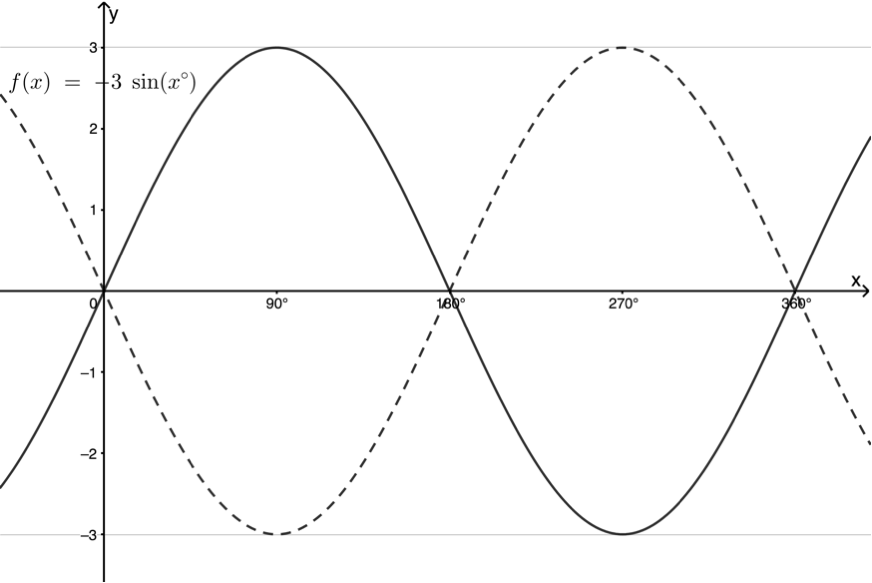Figure 34: Graphs of $\scriptsize f(x)=-3\sin x$ (dotted line) and $\scriptsize g(x)$
3. Sketch the following graphs without using a table of values for the interval $\scriptsize {{0}^{{}^\circ }}\le x\le {{360}^{{}^\circ }}$.
1. $\scriptsize f(x)=\sin x-3$
2. $\scriptsize h(x)=\displaystyle \frac{1}{3}\tan x+1$
4. Given the graphs in Figure 35, answer the following questions.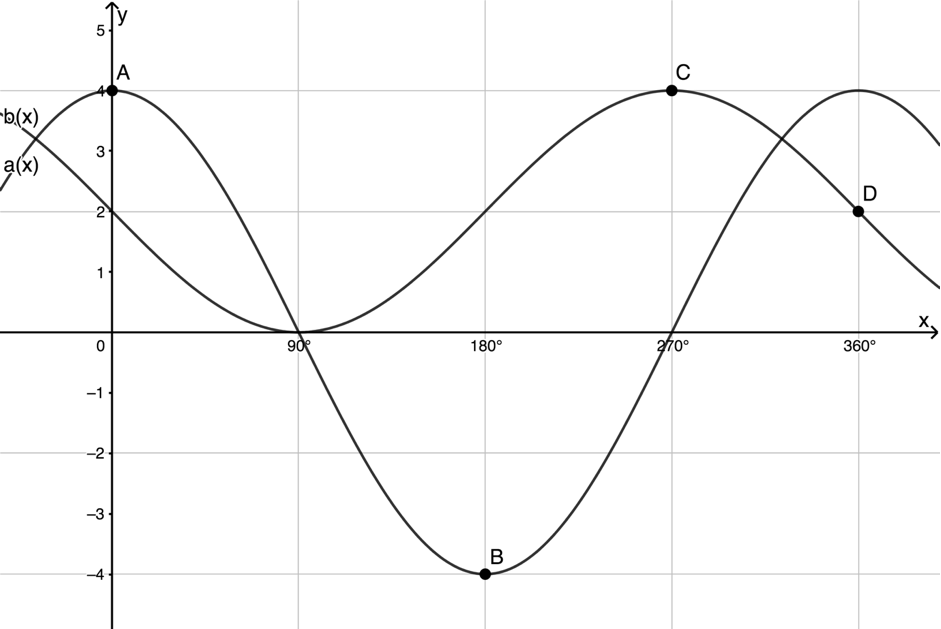Figure 35: Graphs of $\scriptsize a(x)$ and $\scriptsize b(x)$
1. State the coordinates of $\scriptsize A$, $\scriptsize B$, $\scriptsize C$ and $\scriptsize D$.
2. State the domain and range of each function.
3. State the amplitude and period of each function.
4. Evaluate $\scriptsize a({{360}^{{}^\circ }})-b({{360}^{{}^\circ }})$.
5. Evaluate $\scriptsize b({{180}^{{}^\circ }})-a({{180}^{{}^\circ }})$.
6. In the interval $\scriptsize {{0}^{{}^\circ }}\le x\le {{180}^{{}^\circ }}$ for what values of $\scriptsize x$ is $\scriptsize a(x) \gt b(x)$?

The full solutions are at the end of the unit.

# Unit 5: Solutions

### Exercise 5.1

1. .2. .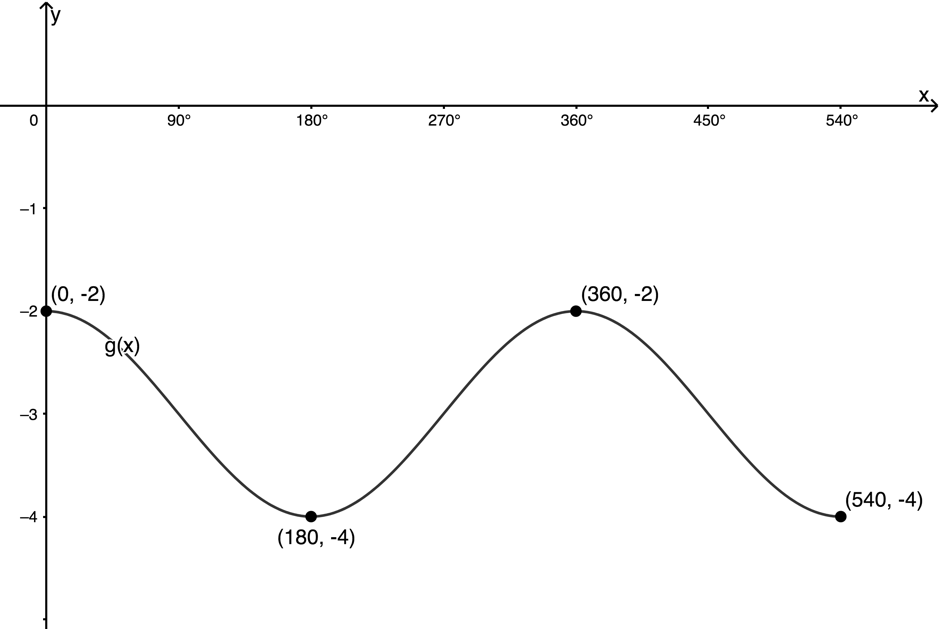Back to Exercise 5.1

### Exercise 5.2

1. $\scriptsize y=-2\sin x-2$. $\scriptsize a=-2$. The amplitude of the graph will be $\scriptsize 2$ and it will be flipped over the x-axis. $\scriptsize q=-2$. The whole graph will be moved two units down. Therefore the ‘middle’ of the graph will now be the line $\scriptsize y=-2$. Therefore, the point normally at $\scriptsize ({{0}^{{}^\circ }},0)$ will move to $\scriptsize ({{0}^{{}^\circ }},-2)$. The point normally at $\scriptsize ({{90}^{{}^\circ }},1)$ will move to $\scriptsize ({{90}^{{}^\circ }},-4)$. The point normally at $\scriptsize ({{180}^{{}^\circ }},0)$ will move to $\scriptsize ({{180}^{{}^\circ }},-2)$.2. $\scriptsize y=-\displaystyle \frac{1}{2}\cos x+1$. $\scriptsize a=-\displaystyle \frac{1}{2}$. The amplitude of the graph will be $\scriptsize \displaystyle \frac{1}{2}$ and it will be flipped over the x-axis. $\scriptsize q=1$. The whole graph will be moved one unit up. Therefore the ‘middle’ of the graph will now be the line $\scriptsize y=1$. Therefore, the point normally at $\scriptsize ({{0}^{{}^\circ }},1)$ will move to $\scriptsize ({{0}^{{}^\circ }},\displaystyle \frac{1}{2})$. The point normally at $\scriptsize ({{90}^{{}^\circ }},0)$ will move to $\scriptsize ({{90}^{{}^\circ }},1)$. The point normally at $\scriptsize ({{180}^{{}^\circ }},-1)$ will move to $\scriptsize ({{180}^{{}^\circ }},\displaystyle \frac{3}{2})$.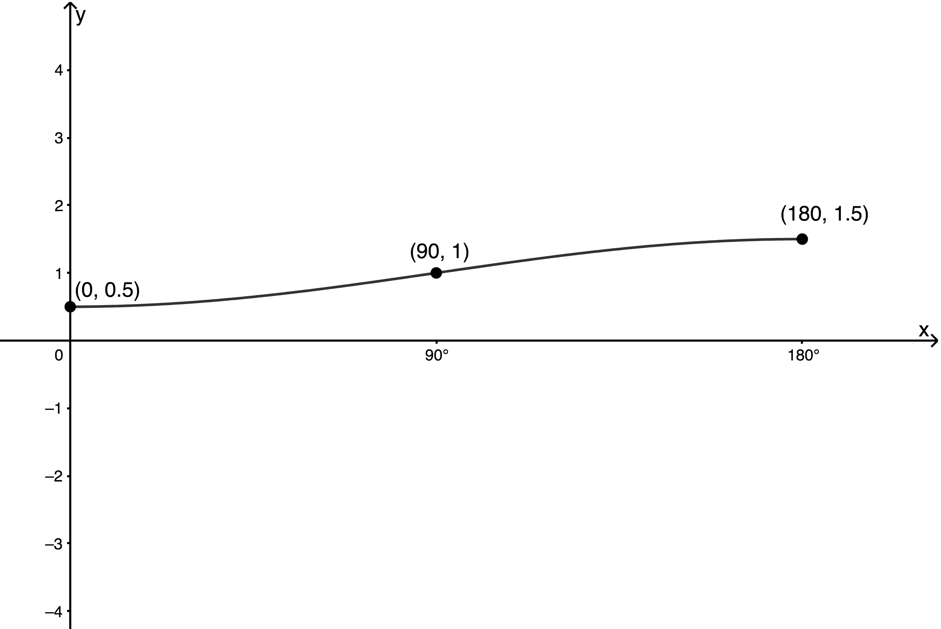Back to Exercise 5.2

### Exercise 5.3

$\scriptsize v(x)=3\tan x-1$. $\scriptsize a=3$ and $\scriptsize q=-1$.

 Original point After $\scriptsize a$ After $\scriptsize q$ $\scriptsize ({{0}^{{}^\circ }},0)$ $\scriptsize ({{0}^{{}^\circ }},0)$ $\scriptsize ({{0}^{{}^\circ }},-1)$ $\scriptsize ({{45}^{{}^\circ }},1)$ $\scriptsize ({{45}^{{}^\circ }},3)$ $\scriptsize ({{45}^{{}^\circ }},2)$ $\scriptsize ({{135}^{{}^\circ }},-1)$ $\scriptsize ({{135}^{{}^\circ }},-3)$ $\scriptsize ({{135}^{{}^\circ }},-4)$ $\scriptsize ({{180}^{{}^\circ }},0)$ $\scriptsize ({{180}^{{}^\circ }},0)$ $\scriptsize ({{180}^{{}^\circ }},-1)$Back to Exercise 5.3

### Exercise 5.4

1. .
1. $\scriptsize \text{A}({{180}^{{}^\circ }},-3)$, $\scriptsize \text{B}({{270}^{{}^\circ }},4)$, $\scriptsize \text{D}({{315}^{{}^\circ }},2)$
2. $\scriptsize f(x)\in [-3,-1]$, $\scriptsize g(x)\in [-2,4]$, $\scriptsize j(x)\in \mathbb{R}$
2. The graph has a maximum turning point where it intercepts the y-axis. Therefore $\scriptsize a \gt 0$. The graph of $\scriptsize y=\cos x$ normally cuts the x-axis at $\scriptsize {{90}^{{}^\circ }}$ which represents the normal ‘middle’ or point of rest of the function. The minimum turning point is at $\scriptsize ({{180}^{{}^\circ }},0.5)$. Therefore, the amplitude of the function is $\scriptsize 2$ and $\scriptsize a=2$.
.
Therefore $\scriptsize y=2\cos x+q$. We can substitute $\scriptsize ({{180}^{{}^\circ }},0.5)$ to find $\scriptsize q$.
\scriptsize \begin{align*}\displaystyle \frac{1}{2} & =2\cos {{180}^{{}^\circ }}+q\\\therefore \displaystyle \frac{1}{2} & =-2+q\\\therefore q & =\displaystyle \frac{5}{2}\end{align*}
$\scriptsize y=2\cos x+\displaystyle \frac{5}{2}$
3. The shape of the function is not the same as the basic tangent function. It has been flipped over the x-axis. Therefore, we know that $\scriptsize a \lt 0$.
Normally, there are anchor points at $\scriptsize ({{45}^{{}^\circ }},1)$ and $\scriptsize ({{135}^{{}^\circ }},-1)$. These are $\scriptsize 2$ units apart. The new anchor points at $\scriptsize ({{45}^{{}^\circ }},-2)$ and $\scriptsize ({{135}^{{}^\circ }},0)$ are still $\scriptsize 2$ units apart. Therefore, the graph has not been stretched out. Therefore $\scriptsize a=-1$ and $\scriptsize y=-\tan x+q$.
.
We can solve for $\scriptsize q$ algebraically. Substitute $\scriptsize ({{45}^{{}^\circ }},-2)$.
\scriptsize \begin{align*}-2 & =-\tan {{45}^{{}^\circ }}+q\\\therefore -2 & =-1+q\\\therefore q & =-1\end{align*}

Back to Exercise 5.4

### Unit 5: Assessment

1. It cannot be graph p. This is a graph of the form $\scriptsize y=a\tan x+q$.
It cannot be graph h. This graph has a maximum at $\scriptsize x={{0}^{{}^\circ }}$. Therefore it is of the form $\scriptsize y=a\cos x+q$.
It cannot be graph q. This graph rises to a maximum at $\scriptsize x={{90}^{{}^\circ }}$. Therefore $\scriptsize a \gt 0$.
Graph g represents $\scriptsize y=-\sin x+1$. The graph falls to a minimum at $\scriptsize x={{90}^{{}^\circ }}$. The base sine function has a maximum at $\scriptsize x={{90}^{{}^\circ }}$. Therefore $\scriptsize a \lt 0$. The graph has an amplitude of 1. Hence $\scriptsize a=-1$ Also, the minimum is not $\scriptsize ({{90}^{{}^\circ }},-1)$ but $\scriptsize ({{90}^{{}^\circ }},0)$ indicating that the graph has been shifted up by one unit. Hence, $\scriptsize q=1$.
2. Both graphs have the same amplitude. Therefore the absolute value of $\scriptsize a$ will be the same. $\scriptsize g(x)$ is the same shape as $\scriptsize f(x)$ except that it has been flipped over the x-axis. Therefore it is also of the form $\scriptsize y=a\sin x+q$ but the sign of $\scriptsize a$ will be opposite. $\scriptsize a=-3$ in $\scriptsize f(x)$. Therefore $\scriptsize a=3$ in $\scriptsize g(x)$ and $\scriptsize g(x)=3\sin x$.
3. .
1. $\scriptsize f(x)=\sin x-3$
The amplitude of the graph is $\scriptsize 1$ and the graph is shifted three units down. So the new ‘middle’ of the graph is the line $\scriptsize y=-3$.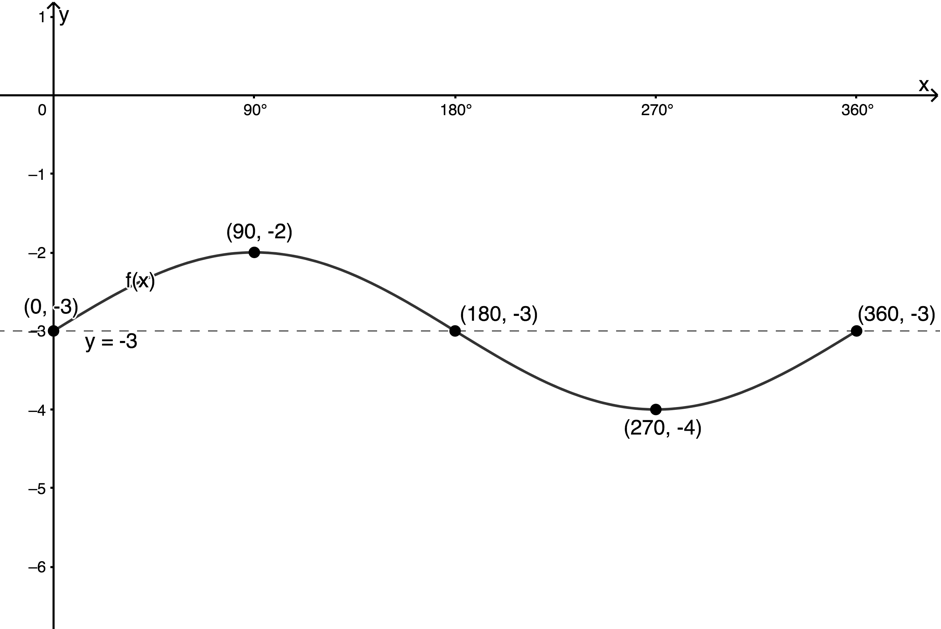2. $\scriptsize h(x)=\displaystyle \frac{1}{3}\tan x+1$
$\scriptsize a=\displaystyle \frac{1}{3}$ so the graph is going to be squashed vertically. The period has not changed so the asymptotes remain the same as the base tangent function. The whole graph is shifted one unit up. The anchor points will be transformed as follows.
 Original point After $\scriptsize a$ After $\scriptsize q$ $\scriptsize ({{0}^{{}^\circ }},0)$ $\scriptsize ({{0}^{{}^\circ }},0)$ $\scriptsize ({{0}^{{}^\circ }},1)$ $\scriptsize ({{45}^{{}^\circ }},1)$ $\scriptsize ({{45}^{{}^\circ }},\displaystyle \frac{1}{3})$ $\scriptsize ({{45}^{{}^\circ }},\displaystyle \frac{4}{3})$ $\scriptsize ({{135}^{{}^\circ }},-1)$ $\scriptsize ({{135}^{{}^\circ }},-\displaystyle \frac{1}{3})$ $\scriptsize ({{135}^{{}^\circ }},\displaystyle \frac{2}{3})$ $\scriptsize ({{180}^{{}^\circ }},0)$ $\scriptsize ({{180}^{{}^\circ }},0)$ $\scriptsize ({{180}^{{}^\circ }},1)$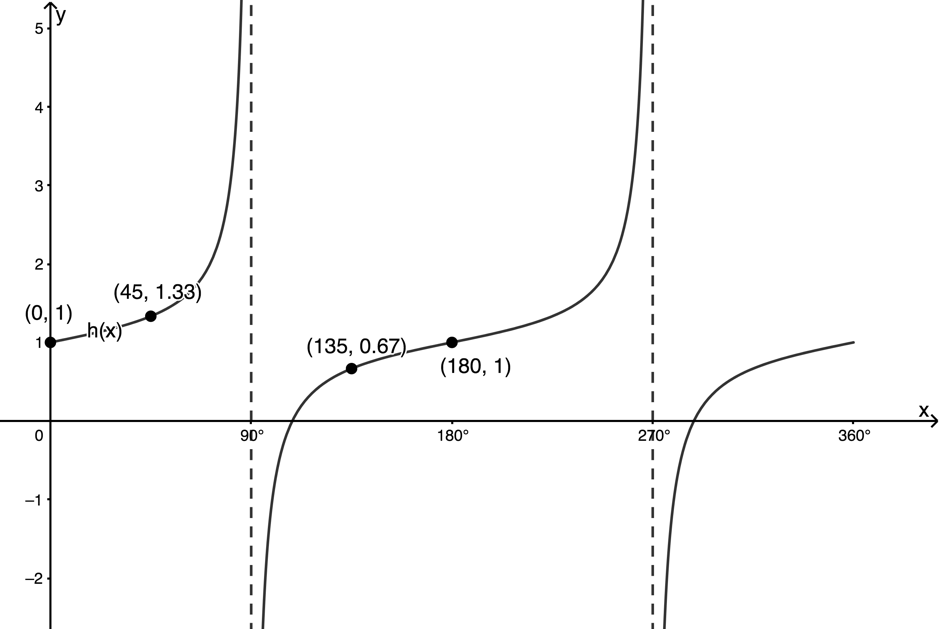4. .
1. $\scriptsize \text{A}({{0}^{{}^\circ }},4)$
$\scriptsize \text{B}({{180}^{{}^\circ }},-4)$
$\scriptsize \text{C}({{270}^{{}^\circ }},4)$
$\scriptsize \text{D}({{360}^{{}^\circ }},2)$
2. $\scriptsize a(x)$: Domain $\scriptsize x\in \mathbb{R}$ ; Range $\scriptsize a(x)\in [-4,4]$
$\scriptsize b(x)$: Domain $\scriptsize x\in \mathbb{R}$ ; Range $\scriptsize b(x)\in [0,4]$
3. $\scriptsize a(x)$: Amplitude is $\scriptsize 4$; period is $\scriptsize {{360}^{{}^\circ }}$
$\scriptsize b(x)$: Amplitude is $\scriptsize 2$; period is $\scriptsize {{360}^{{}^\circ }}$
4. $\scriptsize a({{360}^{{}^\circ }})-b({{360}^{{}^\circ }})=2$
5. $\scriptsize b({{180}^{{}^\circ }})-a({{180}^{{}^\circ }})=6$
6. $\scriptsize x\in ({{0}^{{}^\circ }},{{90}^{{}^\circ }})$

Back to the Unit 5: Assessment